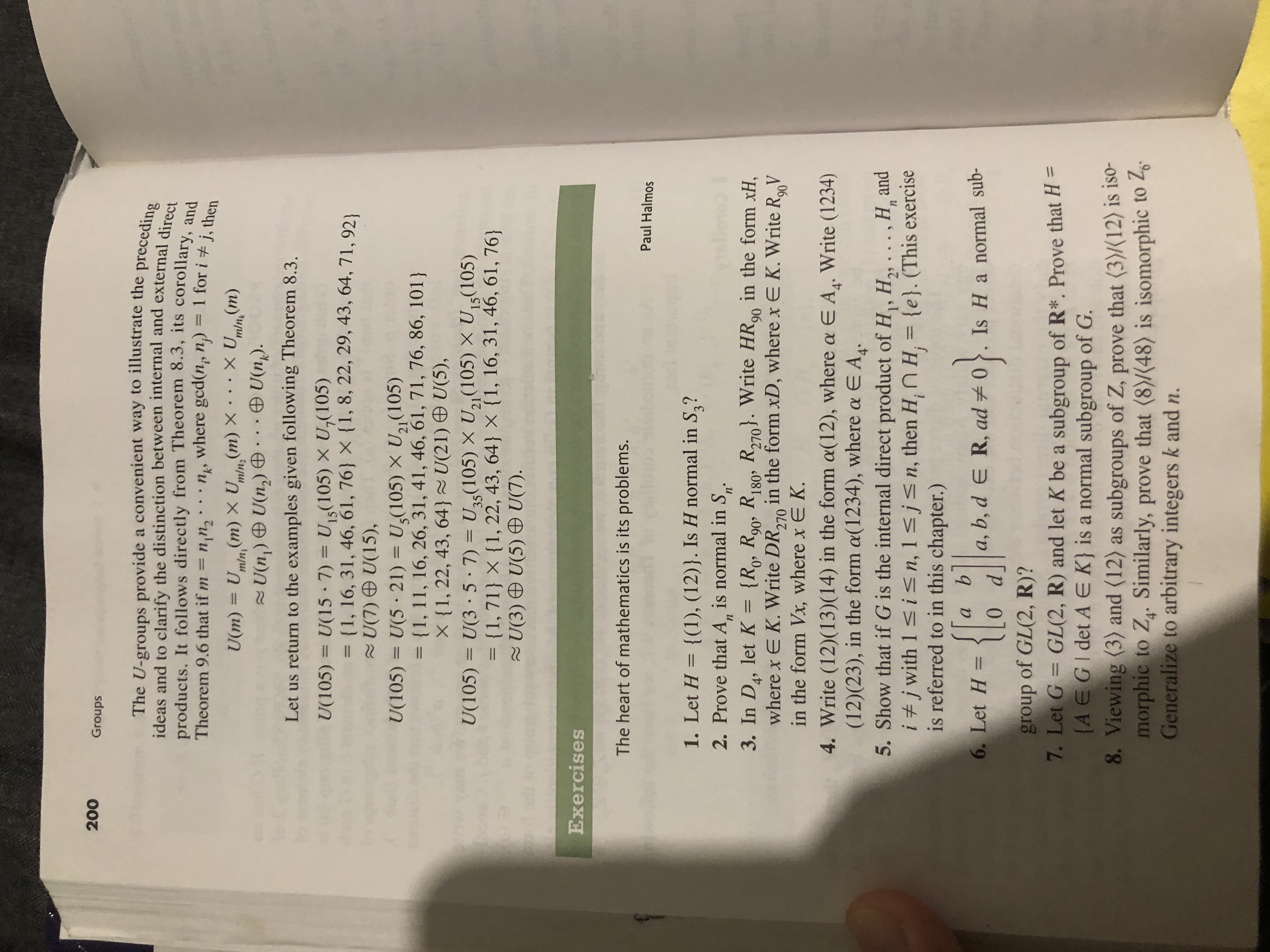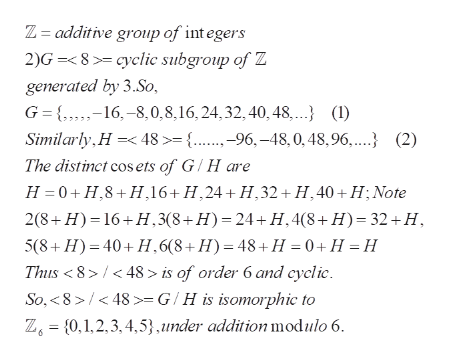# 200GroupsThe U-groups provide a convenient way to illustrate the precedingideas and to clarify the distinction between internal and external directproducts. It follows directly from Theorem 8.3, its corollary, andTheorem 9.6 that if m= nn2where gcd(n, n) =1 for i # j, thenXU(m)(m) X U(m) Xm/nU(m) = UU(n) U(n)mlnminzU(n).Let us return to the examples given following Theorem 8.3U15(105) X U,(105)U(105)U(15 7)= {1, 16, 31, 46, 61, 76 X {1, 8, 22, 29, 43, 64, 71, 92U(7) U(15),U,(105) X U21 (105)U(105)U(5 21)= {1, 11, 16, 26, 31, 41, 46, 61, 71, 76, 86, 101)X {1, 22, 43, 64 U(21) U(5),U(3 5 7)= {1, 71} X {1, 22, 43, 64} X {1, 16, 31, 46, 61, 76}2U(3 ) U(5) U(7).U5(105) X U2 (105) X U5(105)U(105)ExercisesThe heart of mathematics is its problems.Paul Halmos1. Let H = {(1), (12)}. Is H normal in S,?2. Prove that A, is normal in Sп'n3. In D, let Kwhere x E K. Write DR, in the form xD, where x E K. Write RVin the form Vx, where x E K{Ro, R90, R180 R270. Write HR9 in the form xH=,2704. Write (12)(13)(14) in the form a(12), where a E A. Write (1234)(12)(23), in the form a(1234), where a E A.5. Show that if G is the internal direct product of H, H2, ., H andi #j with 1 s in, 1 sjsn, then H, n H, {e}. (This exerciseis referred to in this chapter.)aa, b, d e R, ad 0.Is Ha normal sub-6. Let H =group of GL(2, R)?7. Let G GL(2, R) and let K be a subgroup of R*. Prove that H =(A EGI det A E K} is a normal subgroup of G.8. Viewing (3) and (12) as subgroups of Z, prove that (3)(12) is iso-morphic to Z. Similarly, prove that (8)/(48) is isomorphic to 2Generalize to arbitrary integers k and n.

Question
24 views

8.help_outlineImage Transcriptionclose200 Groups The U-groups provide a convenient way to illustrate the preceding ideas and to clarify the distinction between internal and external direct products. It follows directly from Theorem 8.3, its corollary, and Theorem 9.6 that if m= nn2 where gcd(n, n) = 1 for i # j, then XU(m) (m) X U(m) X m/n U(m) = U U(n) U(n) mln minz U(n). Let us return to the examples given following Theorem 8.3 U15(105) X U,(105) U(105) U(15 7) = {1, 16, 31, 46, 61, 76 X {1, 8, 22, 29, 43, 64, 71, 92 U(7) U(15), U,(105) X U21 (105) U(105) U(5 21) = {1, 11, 16, 26, 31, 41, 46, 61, 71, 76, 86, 101) X {1, 22, 43, 64 U(21) U(5), U(3 5 7) = {1, 71} X {1, 22, 43, 64} X {1, 16, 31, 46, 61, 76} 2U(3 ) U(5) U(7). U5(105) X U2 (105) X U5(105) U(105) Exercises The heart of mathematics is its problems. Paul Halmos 1. Let H = {(1), (12)}. Is H normal in S,? 2. Prove that A, is normal in S п' n 3. In D, let K where x E K. Write DR, in the form xD, where x E K. Write RV in the form Vx, where x E K {Ro, R90, R180 R270. Write HR9 in the form xH = , 270 4. Write (12)(13)(14) in the form a(12), where a E A. Write (1234) (12)(23), in the form a(1234), where a E A. 5. Show that if G is the internal direct product of H, H2, ., H and i #j with 1 s in, 1 sjsn, then H, n H, {e}. (This exercise is referred to in this chapter.) a a, b, d e R, ad 0.Is Ha normal sub- 6. Let H = group of GL(2, R)? 7. Let G GL(2, R) and let K be a subgroup of R*. Prove that H = (A EGI det A E K} is a normal subgroup of G. 8. Viewing (3) and (12) as subgroups of Z, prove that (3)(12) is iso- morphic to Z. Similarly, prove that (8)/(48) is isomorphic to 2 Generalize to arbitrary integers k and n. fullscreen
check_circle

Step 1

Problem concerns quotients G/H , where G and H are subgroups of Z =the additiv group of integers

Step 2

1) proving that the quotient group <3>/<12> is isomorphic to Z4 = cyclic group of order 4,

Step 3

2) <8>/<48> is isomorphic to Z6 , the cyclic group of order 6. The ...help_outlineImage TranscriptioncloseZ= additive group of integers 2)G=<8>= cyclic subgroup of Z generated by 3.So, G { 16, 8,0,8,16,24,32,40,48 (1) Similarly H 48>={...-96,-48, 0,48,96,...} (2) The distinct cos ets of G/H are H 0 H,8 H,16+ H,24+ H,32+ H,40+H; Note 2(8+H) 16+H,3(8+H)=24+H, 4(8+H)=32+H 5(8+H) 40+H.6(8+H)= 48+H 0+ H = H_ Thus <8>< 48> is of order 6 and cyclic So,8>/<48>= G/H is isomorphic to Z= {0,1,2,3,4,5} ,under addition modulo 6. fullscreen

### Want to see the full answer?

See Solution

#### Want to see this answer and more?

Solutions are written by subject experts who are available 24/7. Questions are typically answered within 1 hour.*

See Solution
*Response times may vary by subject and question.
Tagged in

### Math